PHYSICS Mass UNIVERSE UA. Kong Gravitational Force UB. Sun UC. Earth UE. Black Hole UF. Life of Universe UG. Properties of Universe UH. Kong Matter and Kong Energy UH. KONG MATTER AND KONG ENERGY 1. Kong Matter 2. Kong Energy 3. Forming of Mass 4. Rotating Universe 5. Expanding Universe INTRODUCTION   This chapter discusses some interesting topics on the mysteries of Universe, which are the Kong matter and Kong Energy. Kong matter refers to the dark matter that plays important role in ‘stuffing’ the Universe. Kong Energy refers to the dark energy which plays important role in dealing with objects in the Universe.   In this chapter, we will discuss their important roles in this Universe. Some phenomena such as gravitational lensing, mass formation and rotation of matters will be discussed.     OBJECTIVES   1)      To introduce and illustrate the Kong matter 2)      To explain the gravitational lensing due to the Kong matter. 3)      To introduce the Kong energy. 4)      To explain and describe the mass formation and rotation of matters. UH.1.0        KONG MATTER   From chapter “Kong Atom Model”, we understand that mass possesses magnetic and electric (M&E) field, the volumetric of atom is determined by the M&E field. Also from chapter “Fundamental of Physics”, we derived that mass is a function of charge, magnetic field and time. Therefore, we can conclude that matter is inter-related with M&E field.   From chapter “Properties of Universe”, it described that since the beginning of Universe, the Universe obtains a volume which is contributed by the created mass. The whole space of Universe is filled with M&E field and the boundary of Universe is determined by the lowest superimposed quantized magnetic field.   As M&E field is the ingredients for matter and the M&E field fills up the whole universe; we can imply that the ‘empty’ space in the Universe constitutes to the Kong matter. Kong matter is invisible but exists as it is formed by the M&E field which cannot be seen.   The Kong matters play very important role for the followings, a)      as the travel medium for electromagnetic wave, b)      the quantized energy level of electrons, c)      the home for loose electrons, d)      rotation of matters in the Universe, e)      complement to Kong energy     UH.1.1        Mesh of Kong Matter   The existence of M&E field in the space forms the mesh for Kong matter. The mesh is suggested in figure UH.1.1 below,Figure UH.1.1   Figure UH.1.1 gives a non-scale illustration for the mesh of Kong matter, the outermost edge is the boundary of Universe. Generally, for the M&E field in empty space, the M&E components are nearly perpendicular to each other. Empty space refers to Kong matter, while non-empty refers to object.   At certain location of the mesh where objects exist, the M&E fields of objects are non-perpendicular, which is quite similar to atoms. Objects are such as stars, black holes and planets. Although black hole is said cannot be seen, it possesses the properties of an object where its M&E field is not perpendicular.   The different and spherical size of mesh in figure UH.1.1 illustrates the existence of objects at certain location. The M&E field of objects may not necessary to be spherical, but the density of M&E field is higher than empty space.   Superposition of M&E field between the Kong matters and objects produces different pattern of M&E field which is non-perpendicular, depending on the strength of the objects. This is quite similar to a glass where the M&E field within the glass medium is different from outside as described in chapter “Behaviors of Photon”.   Generally, we can represent the difference on the pattern of M&E field mesh by using refraction index. When light travels through a mesh with different refraction index, the light will be slowed down and bent. The bending angle depends on the strength of M&E field, for example, the M&E field within diamond is stronger than glass, where the refraction index of diamond is higher than glass.   Different sizes of objects possess different strength and pattern of M&E field. For an object that possesses higher charge density, its M&E field is stronger, the refraction index is stronger and the affected volume is greater.   At certain circumstance, if an object is opaque, light will be absorbed. The light energy is transferred to the electrons of the object; the electrons are either vibrating in the object or escape from the object.     UH.1.2        Gravitational Lensing   From chapter “Behaviors of Photon”, it describes about the phenomena on refraction and diffraction of light and the energy level of light when passes through a non-perpendicular M&E field. Here, we will adopt the same phenomenon in describing gravitational lensing.   Also from chapter “Energy of Photon”, it derives on the kinetic and potential energy of photon. When a photon travels in the speed of light, it is mass-less. How can a mass-less photon be attracted by the mass-dependent gravitational force?   In the Universe, varies kind of objects appear and scatter around. When light passes through near to an object, light undergoes diffraction, depending on the strength of M&E field of that object. This phenomenon is called gravitational lensing.   Black hole is an object which possesses very strong charge as describe in chapter “Black Hole”. The core of the black hole is the iron ashes at very high positive charge. The strength of the M&E field of black hole is very strong, the affected volume is huge and the refraction index is high.   Gravitational lensing happens to black hole. When light passes through near a black hole, the light will be diffracted. The speed of light reduces and bent inward to the black hole. The bending angle is depends on the refraction index and the frequency of light. If the light possesses higher energy, the bending angle is smaller and light can escape from the black hole to the space. If the light possesses lower energy, the bending angle is bigger and light may be absorbed into the event horizon of the black hole. This phenomenon is quite similar to the diffraction of light through a thin film, where light is diffracted to wider angle when passes through an atom in the thin film. UH.2.0        KONG ENERGY   In this Universe, many activities are governed by one kind of energy. It is given by the name ‘Kong energy’, which is equivalent to dark energy. Kong energy produces certain virtual forces to ‘do’ work in this Universe, which adheres to the principle of energy conservation.   To simplify the mystery about Kong energy, Kong energy refers to the effects which caused by the change of M&E field in the Universe, adhering to the natural law of Faraday’s and Ampere-Maxwell’s law of induction. The work done by the Kong energy is a kind of energy transformation.   The effect of Faraday’s law of induction is greatly contributing to the Kong energy, where the change in magnetic field will induce an electric field. The effect of Ampere-Maxwell’s law of induction is yet to be observed. But Ampere’s law of induction greatly affects the shape of universe, where the elongated magnetic field produced by rotating current contributes to the flat Universe.   These two natural laws that affect the activities in the Universe are grouped under the effects of Kong energy. UH.3.0       FORMING OF MASS   Under the influence of Kong energy, mass are created during BB. Mass formation process is one of the effects of Kong energy.   From chapter “Properties of Universe”, we understand that mass is created during big bang. Right before the Universe ends, it contains gamma rays, which is the kinetic-time-energy. The contraction of Universe into a point converts the kinetic-time-energy to potential-time-energy. This process is similar to pair production process.   The Universe then expands at the speed of light at time zero, the positrons and electrons are produced. Both particles and anti-particles are spinning at the speed of light. This pair production process has no time delay as it happens at the speed of light. The creation of particles produces the volume and space of the Universe.   The created positrons and electrons are spinning at the speed of light and without reactive magnetic gauss line. Reactive gauss line is required for chemical bonding.   From chapter “Fundamental of Physics” regarding the dissection on the dimension of energy, energy consists of the four fundamental ingredients as follow,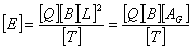… eq. UH.3.1 where   [E] = Dimension for Energy             [Q] = Dimension for charge             [B] = Dimension for magnetic field             [L] = Dimension for length             [T] = Dimension for time             [AG] = Gaussian surface   At T = 0, the volume is zero, there is no information on the Energy. At T = δt, the Gaussian surface is small, the produced charge and magnetic field can be huge.   The Gaussian surface is used to interpret one of the dimensions for energy because energy transformation within the Gaussian volume is conserved. To relate the energy to its dimensions, by adding a constant into eq. UH.3.1, we can write as follow,… eq. UH.3.2   Differentiation on eq. UH.3.2 with respect to time, we obtain,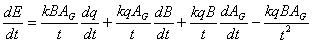… eq. UH.3.3   As the Universe expands at the speed of light after time zero, the change of Gaussian surface over time is great, the charge remains no change and the magnetic field reduces drastically.   From eq. UH.3.3, for conservation of energy, the change in energy is zero. The change in net charge is also zero. We arrange to obtain the changing rate of magnetic field as follow,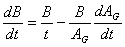… eq. UH.3.4   At time after zero, where t = 0 + δt, the value of t ≈ AG, therefore, rearrange eq. UH.3.4, we obtain,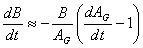… eq. UH.3.5   Also during the earlier time, dAG/dt >> 1, eq. UH.3.5 reduces to,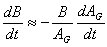… eq. UH.3.6   As the change of Gaussian surface is greatest at the earliest stage of BB, the change of magnetic field is also the greatest. The negative sign shows that the changing rate of magnetic field is decreasing.   The decrease of magnetic field induces and enriches the positrons to become bigger particles, where the component of magnetic field is temporarily ‘stored’ by positrons. The forming of bigger particles is to resist the change in magnetic field. The positrons are agglomerated, lumped and restructured to form bigger particles or atoms, which contain higher M&E field component with higher quantized magnetic gauss line. The magnetic gauss line produced is the reactive magnetic gauss line, which is possible for chemical bonding with other particles.   Part of the mass is contributed by electric component and part of the mass is contributed by magnetic component. The formation of mass must achieve certain quantum mechanics in order to be stable.   As the mass formation process creates a force to resist the expansion, the expansion rate of Universe is decreasing. The expansion rate will be discussed later. The size of atom formed depends on the expansion rate. Lower expansion rate produces atom at lower atomic number and mass number. Hence, atom formed at earlier stage possesses higher mass number and atomic number and vice versa at later stage.   Surrounding electrons are then attracted by the atom and form a complete atom; the M&E fields of the atom are the home for electrons.   Mass formation process continues. As the expansion rate is reducing, the period for mass formation at certain quantum mechanics is longer. Therefore, the quantity of atoms at lower mass number is more than the atoms at higher mass number. Due to this reason, the quantity of hydrogen atoms is the highest in the Universe.   In this mass formation process, the anti-particles of electrons, which are the positrons, are grown by the magnetic field to become atoms, while electrons live in the magnetic gauss line of atoms. This condition causes the matters are more than anti-matters. The Kong energy induces the mass formation process during the early stage of Universe. UH.4.0        ROTATING UNIVERSE   Under the influence of Kong energy, the Universe is rotating to its center of rotation. The rotating Universe is one of the effects of Kong energy.   After the mass formation process, when the last hydrogen atom is formed, the remaining positrons are unable to achieve certain quantum mechanics due to the low expansion rate of Universe. The remaining positrons annihilate with the existing electrons and produce gamma rays.   The gamma rays then ionize the atoms. Electrons absorb the energy from gamma rays and escape from atoms. The energy is transferred from the electromagnetic wave to the kinetic energy of electrons. The escape electrons live in the magnetic gauss line of Universe instead of in the atoms.   This interaction continues between wave and electrons while the Universe is expanding and temperature is reducing. The electromagnetic wave radiation is corresponding to the temperature of Universe.   When Universe continues to expand, the magnetic field is reducing. At this stage after ionization, mass is not able to be produced. The Universe needs to create a force to resist the change in magnetic field.   In accordance to the Faraday’s law of induction, as shown below,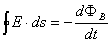… eq. UH.4.1   Faraday’s law shows that a changing magnetic field produces an electric field. Figure UH.4.1 shows the relationship for eq. UH.4.1. Figure UH.4.1 shows the top view of the Universe. The magnetic field is pointing outwards from the section. When the magnetic field is reducing, an electric force will be created to resist the change in magnetic field. The magnetic field across the horizontal cross sectional surface, Ac, is the magnetic flux.Figure UH.4.1   From eq. UH.4.1, the magnetic flux is given by, ФB = B · Ac                                                                             … eq. UH.4.2   From figure UH.4.1, the vector of magnetic and Ac is pointing outward from paper or pointing up at the front view of Universe. Therefore, eq. UH.4.2 becomes, ФB = B Ac                                                                                … eq. UH.4.3   Differentiate eq. UH.4.4 with respect to time to obtain the changing rate of magnetic flux,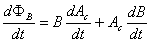… eq. UH.4.4   During the early time, the changing rate of magnetic field is the greatest, where eq. UH.3.6 is applicable. Substitute eq. UH.3.6 into eq. UH.4.4, we obtain,…eq. UH.4.5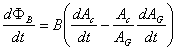…eq. UH.4.6   Also during the early time of BB, the shape of Universe is almost spherical. Hence, we can write the followings, AG = 4πr3                                                                                …eq. UH.4.7 Ac = πr2                                                                                   …eq. UH.4.8   Differentiate eq. UH.4.7 and UH.4.8 with respect to time, we obtain,…eq. UH.4.9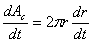…eq. UH.4.10   Substitute eq. UH.4.7, UH.4.8, UH.4.9 and UH.4.10 into eq. UH.4.6, we obtain,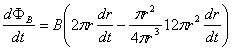…eq. UH.4.11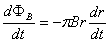…eq. UH.4.12   The magnetic flux is depending on the expansion rate. The negative sign in eq. UH.4.12 shows that the magnetic flux is decreasing.   Substitute eq. UH.4.12 into eq. UH.4.1, we obtain,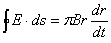… eq. UH.4.13   The induced electric field is circular, therefore, the integration on the left side of eq. UH.4.13 becomes,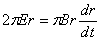… eq. UH.4.14… eq. UH.4.15   The induced electric field during the early time of BB is depending on the expansion rate of Universe. The positive sign in eq. UH.4.15 shows that the induced electric field is in anti-clockwise direction. The decreasing magnetic flux induces a circular electric field in anti-clockwise.   The circular electric field creates a force to push the charged atoms to rotate in the Universe. The Universe is rotating to its center of rotation. The center of rotation is the center of magnetic field and also the point of expansion and contraction of Universe.   The continuous reduction in magnetic flux induces a continuous circular electric field; this creates a continuous force to increase the angular velocity of rotation of Universe. The objects rotate faster and faster around the center of rotation. This energy refers to the Kong energy which causes the rotation of Universe and increases the rotation speed. UH.5.0        EXPANDING UNIVERSE   It is observed that the objects around us are moving away, this implies that the Universe is expanding. The expansion rate must be less than the speed of light; otherwise, light from another star or galaxy will never reach us.   The Kong energy also plays an important role in the expansion of Universe. The Universe is expanding at the speed of light at time zero of big bang, in relation with the remaining gamma rays at the ending. The expansion rate is then decreasing to the speed lower than the speed of light, due to the resistance created by Kong energy.   The Universe expands in radial direction at the equator, from spherical until it becomes a disk as it is today. Figure UH.5.1 below illustrates the front view and top view for the mesh of Kong matters.Figure UH.5.1   The Gaussian surface in figure UH.5.1 is the boundary of Universe and Ac is the horizontal cross sectional area on the equator.   When Universe expands, magnetic flux is decreasing. The decrease in magnetic flux induces a circular electric field that pushes the matters to rotate in anti-clockwise direction around the center of rotation, which is shown in figure UH.4.1.   The decrease in magnetic flux is caused by two reasons. First reason is due to the expansion as shown in eq. UH.3.6. Second is due to the reduction of mass.   As the expansion continues, the electric field will be induced all the time. The induced electric field accelerates the angular velocity of objects in the Universe.     UH.5.1        Expansion Rate   From highlighted portion in figure UH.5.1, we can write an equation for the relation of radial and lateral components as follow,   s = rθ                                                                                      … eq. UH.5.1   Differentiate eq. UH.5.1 with respect to time, we obtain,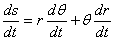… eq. UH.5.2 where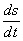= lateral expansion rate or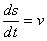= lateral velocity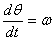= angular velocity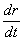= radial velocity or the expansion rate   Rearrange eq. UH.5.2 to obtain the expansion rate as follow,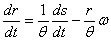… eq. UH.5.3   From eq. UH.5.3, the expansion rate shows that during the early stage where the angular velocity, ω, is approaching zero, the radial expansion rate is proportion to the lateral expansion rate, which is the highest. When the angular velocity increases, the radial expansion rate decreases. When the radial expansion rate reduces to zero due to deceleration, eq, UH.5.3 becomes,   v=rω                                                                                       … eq. UH.5.4   The lateral velocity is the radius of Universe multiplies with the angular velocity. This radius is the biggest size of Universe.   To show that the expansion is decreasing, we apply second order differentiation to eq. UH.5.2, we obtain,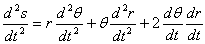… eq. UH.5.5 where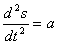= lateral acceleration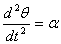= angular acceleration= radial acceleration or the expansion acceleration   Rearrange eq. UH.5.5 to obtain the expansion acceleration,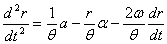… eq. UH.5.6   For the assumption that if the charged particles are distributed uniformly in space, the resultant lateral force is zero, the lateral acceleration is zero. Hence, eq. UH.5.6 reduces to,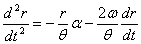… eq. UH.5.7   The angular acceleration is caused by the induced circular electric field which is in anti-clockwise direction. The angular velocity is always in anti-clockwise direction and the radial velocity is always in positive direction. Hence, the expansion acceleration is always negative, which is deceleration.   The expansion rate or radial velocity is reducing from the speed of light to zero, but the angular velocity of objects is increasing as long as the circular electric field is induced by the decreasing magnetic flux.   When the expansion rate reaches zero, the Universe then begins to contract due to the continuous decreasing magnetic field, which caused by the reduction of mass.     UH.5.2        Contraction Rate   The contraction of Universe is caused by the reduction of mass. Reduction of mass decreases the strength of magnetic field. Reduction of the cross sectional area, Ac, also decreases the magnetic flux, the change in magnetic flux induces a circular electric field as shown in eq. UH.4.1. The induced electric field contributes to accelerate the angular movement of matters.   From eq. UH.5.6, the contraction rate is,… eq. UH.5.8   Assume that the lateral force is balance due to the uniform distribution of charged particles. Eq. UH.5.8 reduces to eq. UG.5.9 as follow,… eq. UH.5.9   From eq. UG.5.9, the induced circular electric field exacts a force to accelerate the angular velocity. The rotation of matters becomes faster. The angular velocity and acceleration are positive due to anti-clockwise direction. The radial expansion velocity is negative due to contraction. The resultant expansion acceleration has negative value which is acceleration to the contraction.   The Universe contracts into a point and revive. DISCUSSIONS AND CONCLUSIONS   The magnetic and electric (M&E) field that stuffing the whole empty space in the Universe is the Kong matter. Charge and magnetic field are the ingredients of mass or matter; therefore, the Kong matter refers to the M&E field in the Universe.   Superposition of the M&E field for objects and Kong matter produces different pattern and strength of M&E fields at certain location in the Universe, which can be related to refraction index. The effect of gravitational lensing is due to behavior of light when passes through space with different refraction index.   Kong energy refers to the effects which caused by the change of M&E field in the Universe, adhering to the natural law of Faraday’s and Ampere-Maxwell’s law of induction. The mass formation, rotation of Universe and expansion of Universe are few effects that caused by Kong energy.   During the mass formation process, atoms are created when positrons are lumped, agglomerated and restructured together due to the rapid change in magnetic field. The atoms created possess reactive magnetic gauss line which able to perform chemical bonding. Electrons live in the magnetic gauss line of atoms. Heavier atoms are formed in the early stage and lighter atoms are formed in the later stage of Big Bang, due to the different changing rate of magnetic field. Lighter atoms in quantity are more than heavier atoms because the formation period for lighter atoms is longer.   The rotation of object in the Universe is caused by the reduction of magnetic field which adheres to the faraday’s law of induction. The reduction in magnetic flux induces a circular electric field that pushes the objects to rotate in acceleration.   The Universe is expanding at the rate slower than the speed of light. The Universe expands from the speed of light to zero speed and then contracts. The deceleration of expansion and acceleration of contraction is caused by the changing magnetic field in the Universe, which is the one of the effects of Kong energy.

This website is originated on 15-Mar-2007,

updated on 4-Jan-2009.